# N8PPQ

## My Personal Site

### Graphing Calculators and the Regression Technique

A modern textbook problem goes like this: students are provided a real world manifestation of a functional relationship, complete with a collection of data. A graphing calculator is used to plot data and to impose a curve through the points. The "regression" technique is generally seen by the student to be a sort of proof that the particular relationship exists between the variables.

Having arrived at the desired and presumably concluding step, students are satisfied with their accomplishment (or at least relieved) and resist further inspection. They avoid the paradox that other students have obtained equally professional looking displays while using the ‘wrong’ functional choice; a cubic rather than a quadratic regression for example. The teacher or the text is expected to provide a judgment. An explanation is actually required.

 I use an example of my own. I tell the class that I had stuck my head into the sink at home to get a drink and noticed an interesting curve. (This line guarantees a chuckle, but I want to apply math to ordinary situations). I show this picture on the overhead and point out that water falls in a laminar column of decreasing radius. I suggest that the vertical edge looks somewhat like a parabola, and that we might use quadratic regression to chart it on a calculator. The idea is acceptable. The curve is certainly not linear, and students are familiar with parabolas.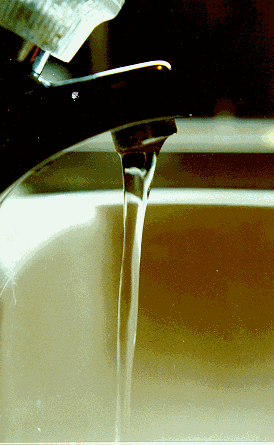The varying radius of tap water would be difficult to measure directly. I recommend that we use a photograph. I also advise us to use syrup rather than water. I ask them to speculate during the experiment as to why syrup might be better.

As a chosen student empties syrup into a bowl, I snap a half dozen pictures with a digital camera and distribute printouts to small groups. Each student fills in a table relating distance fallen to radius.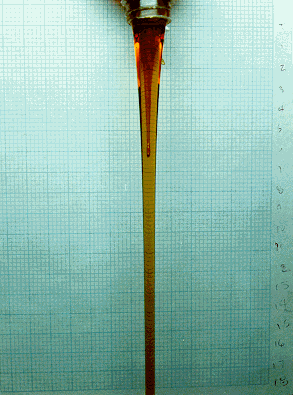I use graph paper as a backdrop to eliminate some of the measurement problems. As you can see, the boxes seem to get smaller near the bottom, where they are farther from the camera. I keep the bottle close to the backdrop to reduce other errors resulting from the angle of vision. After tabling data, students chart the points on a graphing calculator and impose a second order polynomial curve through them. They are then sufficiently pleased/relieved. I continue by presenting tabled measurements and a computer graph of my own results. Via inquiry, we decide that our plots are inverted representations because our conventional machines place positive numbers in the first quadrant. Our curves are also bumpy, illuminating the difficulty of measuring this way.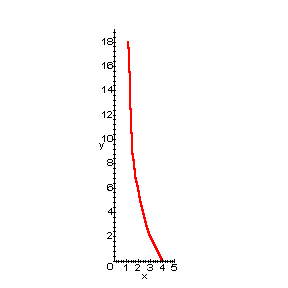I show my tabulated data on the overhead:

 Distance Diameter Radius 0 8.1 4.05 1 7.0 3.50 2 6.0 3.00 3 5.3 2.65 4 4.8 2.40 5 4.4 2.20 6 4.0 2.00 7 3.6 1.80 8 3.3 1.65 9 3.1 1.55 10 3.1 1.55 11 2.9 1.45 12 2.9 1.45 13 2.7 1.35 14 2.7 1.35 15 2.7 1.35 16 2.6 1.30 17 2.5 1.25 18 2.4 1.20

Selecting three representative points from the list above, I invoke Lagrange's formula for interpolation, producing a quadratic function, which includes the points*.I used Maple to produce the plot shown here, with the curve passing through the selected points. We have a pretty good fit. Selecting other points for the interpolation may have resulted in a more accurate representation.       *I don't expect them to know how to do this yet, but they can see it without threat. This is a radical break from routine, (packaged within the firmware).

I used the same method to look for a polynomial of degree four. I selected points (1.2, 18), (2.4, 4.0), (2.65, 3.0), (3.0, 2.0), (3.5, 1.0). Part of the Maple code is shown below.

 > Lmo:=(x->((x-2.4)*(x-3)*(x-3.5)*(x-4.05))/((1.2-2.4)*(1.2-3)*(1.2-3.5)*(1.2-4.05))); > Lm1:=(x->((x-1.2)*(x-3)*(x-3.5)*(x-4.05))/((2.4-1.2)*(2.4-3)*(2.4-3.5)*(2.4-4.05))); > Lm2:=(x->((x-1.2)*(x-2.4)*(x-3.5)*(x-4.05))/((3-1.2)*(3-2.4)*(3-3.5)*(3-4.05))); > Lm3:=(x->((x-1.2)*(x-2.4)*(x-3)*(x-4.05))/((3.5-1.2)*(3.5-2.4)*(3.5-3)*(3.5-4.05))); > Lm4:=(x->((x-1.2)*(x-2.4)*(x-3)*(x-3.5))/((4.05-1.2)*(4.05-2.4)*(4.05-3)*(4.05-3.5))); > Pm4:=(x->18*Lmo(x)+4*Lm1(x)+2*Lm2(x)+1*L3(x)+.00001*Lm4(x)); > ch1:=plot (Pm4(x), x=0..5): > display ([ch1, ch3]);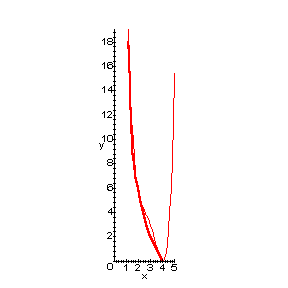Now this curve fits much better. It's not perfect, but there is nothing in the graph to contradict the notion that distance fallen is proportional to the fourth power of the radius, (that is if we admit that all radii greater than the initial one are meaningless). We might claim that evidence shows the direct relationship and point to this display as our proof. But of course that's not right.

So why are we doing it? That is the important question and the essence of these kinds of exercises. It is also the most easily neglected issue - but what should we assess if this point is missed?

I submit to the students that if we need a mathematical model of this particular column of syrup, we have one. We could use a linear model for small sections, but the quadratic fits the whole column better. The fourth degree fits better still, but parabolas are familiar and (hopefully) friendly curves. We could maybe use one for whatever calculations we might need to do with this example.

But we might be able to derive a model for all similar effusions. If we could link radius to distance from the spout in some general way, we may have a better handle on all sorts of situations. I present the following in front of the whole class, (thus modeling modeling).

We need equations in a mathematical model, so we list some. We can assume that the amount of syrup flowing from the spout is equal to the amount flowing past any other location. We will call this quantity "flux" and define it as cross-sectional are a perpendicular to the direction of flow multiplied by the syrup velocity. This flux remains constant everywhere along the column. In math: A0v0 = Axvx . This falls from the property of conservation of mass. I remind them that area has a square term in its formula.

I state that the velocity at any point is equal to the initial velocity, (almost zero at the spout) plus the time traveled multiplied by the acceleration of gravity, which is 32 feet per second, per second, (neglecting air resistance, etc.). Thus: vx = v0 + tg. This added variable, time, has not made our job easier. To avoid it, we can use the energy equations (familiar to high school students). Kinetic energy is defined as 1/2 mass multiplied by the velocity squared (another square term). Potential energy is mass times acceleration of gravity times the relative height.

So, we work through the problem analytically, as a class:

(1) A0v0 = Axvx

from Conservation of Mass

(2) A0 = p r02, Ax = p rx2

from Geometry, and

(3) mgh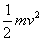from Conservation of Energy. So h is proportional to v2. Now plugging (2) into (1) and simplifying we have

r02 v0 = rx2 vx .

Solving (3) for vx and plugging in above (disregarding the negative root) we have

r02 v0 = rx2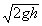.

Solving this relation for h we have

(4) h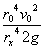.

Letting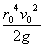= K we have

(5) h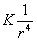.

We see that the distance is actually inversely proportional to the fourth power of the radius. Of course! The radius decreases as the distance increasesI have the students plot this function, using convenient values (i.e. K = 10) to see how similar the curve is to the photograph.      I remind students that the domain of a polynomial curve like our original parabola is boundless, whereas actual radii are only positive. The inverse function is respectfully undefined at r = 0.

Returning to the decision to use syrup, we brainstorm to conclude that syrup 1) tastes better, 2) is easier to photograph, 3) is more viscous than water and would provide a slower initial velocity. The smaller v0 in (4) and resulting smaller K in (5) provides a more noticeable curve for given r0. Syrup also reduces the effect of standing waves, clearly visible in the photographs above. (I don't know what causes the standing waves – but I think it's important for a teacher to leave some questions unanswered.)

I stress that inverse fourth model is still only a good approximation. We have not accounted for the standing waves, air resistance, measurement errors, and more. We can now deal with similar laminar flows in a general way, and what we have may be suitable for most applications, but is still flawed to some degree. As mathematicians, we should endeavor to evaluate that error. (We have not done that in my high school class, but I emphasize the need to do it someday.)

Finally, I ask the students to ponder the usefulness of our discovery. I suggest possible engineering situations where it might be important to predict surface area or volume.

The experiment I outlined here takes 40 minutes to pull off. After witnessing some of the remarkable claims by college students regarding the meaning of the "regression" technique, and the confusion within high school classrooms as to its purpose, I think this is time well spent.

__________________________________